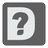# Determinant

Elementary+
English RU

In linear algebra, the determinant is a value associated with a square matrix. It can be computed from the entries of the matrix by a specific arithmetic expression (There are other ways to determine its value as well.)

The determinant of a matrix A is denoted det(A) , det A , or |A| . In the case where the matrix entries are written out in full, the determinant is denoted by surrounding the matrix entries by vertical bars instead of the brackets or parentheses of the matrix.

There are various ways to define the determinant of a square matrix A , i.e. one with the same number of rows and columns. Perhaps the most natural way is expressed in terms of the columns of the matrix. If we write an N×N matrix in terms of its column vectors:

```A = [a1, a2, ..., an]

```

Where there are vectors of size n, then the determinant of A is defined so that:

```det[a1, ..., b*aj+c*v, ..., an] = b*det(A)+c*det[a1, ..., v, ..., an]
det[a1, ..., aj, aj+1, ..., an] = -det[a1, ..., aj+1, aj, ..., an]
det(I) = 1

```

Where b and c are scalars, v is any vector of size N and I is the identity matrix of size N . These properties state that the determinant is an alternating multilinear function of the columns, and they suffice to uniquely calculate the determinant of any square matrix. Provided the underlying scalars form a field (more generally, a commutative ring with unity). Equivalently, the determinant can be expressed as a sum of products of entries of the matrix where each product has N terms and the coefficient of each product is −1 or 1 or 0 according to a given rule: it is a polynomial expression of the matrix entries. This expression grows rapidly with the size of the matrix (an NxN matrix contributes N! terms), so it will first be given explicitly for the case of 2×2 matrices and 3×3 matrices, followed by the rule for arbitrary size matrices, which subsumes these two cases.

Input: A square matrix as a list of lists with integers.

Output: The determinant of the matrix as an integer.

Example:

```checkio([[4,3], [6,3]]) == -6

checkio([[1, 3, 2],
[1, 1, 4],
[2, 2, 1]]) == 14```

How it is used: The determinant is a basis for linear algebra and mathematical software. Linear algebra is used in vector graphics to calculate line paths and shapes. It can also be of use for many optimisation problems along with cryptography (videocipher) and geometry (testing for collinear points).

Precondition:
0 < len(matrix) ≤ 5
all(len(row) == len(matrix) for row in matrix)
all(all(0 ≤ x < 10 for x in row) for row in matrix)

You should be an authorized user in order to see the full description and start solving this mission.21
Settings
Code:
Other:
CheckiO Extensions

CheckiO Extensions allow you to use local files to solve missions. More info in a blog post.

In order to install CheckiO client you'll need installed Python (version at least 3.8)

Install CheckiO Client first:

`pip3 install checkio_client`

`checkio --domain=py config --key=`

Sync solutions into your local folder

`checkio sync`

(in beta testing) Launch local server so your browser can use it and sync solution between local file end extension on the fly. (doesn't work for safari)

`checkio serv -d`

Alternatevly, you can install Chrome extension or FF addon

`checkio install-plugin`
`checkio install-plugin --ff`
`checkio install-plugin --chromium`

Read more here about other functionality that the checkio client provides. Feel free to submit an issue in case of any difficulties.